Buffer Equations and Buffer Capacity in General : Pharmaguideline -->
 Editable Pharmaceutical Documents in MS-Word Format

# Buffer Equations and Buffer Capacity in General

Buffers are characterized by the fact that their pH remains constant, are not affected by dilution, by the addition of small amounts of acids or bases

## Buffer Equation

Buffers are characterized by the fact that their pH remains constant, are not affected by dilution, by the addition of small amounts of acids or bases, and are not affected by prolonged storage of the solution.

The addition of small amounts of acid or base to moderate pH solutions results in absorption by buffer with minimal pH changes. A small number of acids or bases can significantly change the pH of extreme pH solutions, for example, pH 1 has a high acid concentration (0.1 M), and a small amount of acid or base won't change the pH of such a solution. Calculations involving weak acids require a knowledge of the pKa of the acid. It is possible to calculate the pH of the buffer solution by rearranging the equation
• For dielectric constant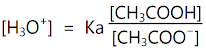equation (1)

A solution's acetic acid concentration can be viewed as the total amount of acid in the solution since acetic acid is not readily ionized. In an alternate form, the term [CH3COO-] may replace the term [Acid], as well as [CH3COOH] for [Salt]. Therefore,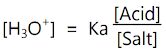equation (2)

Calculating the pH of buffer solutions containing both acid and its conjugate base can be performed by rearranging and rewriting the dissociation constant equation as follows: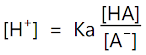equation (3)

The acid concentration [HA] corresponds to its conjugate base concentration [A–]. In logarithmic form, equation (3) is: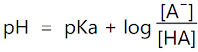equation (4)

i.e.,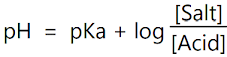The Henderson-Hasselbalch equation (Equation 4) is called this. HA/A–, HA– /A2- or B+ /BOH are examples of conjugate bases that can be used to calculate pH in solutions. Similar to weak acid buffers, weak base buffers can be derived from their corresponding salt equations. So,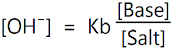equation (5)

Because water (Kw) is an ionic compound composed of H3O+ × OH–

OH– = Kw/H3O+ equation (6)

Equation (6) is derived by substituting value for OH-equation (7)

The logarithm of equation (7) is as follows: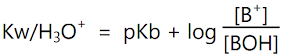equation (8)

i.e.,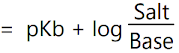Or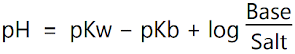equation (9)

Salt, acid, and base are all represented by their molar concentration. A pH of a solution can be calculated using Henderson-Hasselbalch equations and acid and base solutions. A solution with 0.1 M acetic acid and 0.05 M NaOH can be calculated using the Henderson-Hasselbalch equation. When half the acid has been neutralized, then the base and the acid have identical concentrations. Since the logarithmic quotient is 1, pH = pKa.

When acid and conjugate base equilibrium concentrations are present, the Henderson-Hasselbalch equation holds. Solution concentrations of solutions containing not-so-weak acids (or base concentrations that are not so weak) can differ dramatically from the concentrations of the ingredients added to the solution. Dichloroacetic acid (pKa = 1.5) can be substituted for acetic acid to result in pH values of 1.78 due to its ability to dissociate by itself. Due to this, the equilibrium concentration of the conjugate base is 0.0334 M rather than 0.05 M.

When the Henderson-Hasselbalch equation is used to predict pH it can fail to give accurate results, especially if the solution is diluted. Acids with pKa less than 2.5 dissociate too easily, so the Henderson-Hasselbalch equation should be avoided altogether. The Henderson-Hasselbalch equation gives good results with an error of less than 2.5 pKa for solutions above 10 mM and weak acids below pKa ≥ 2.5. Similarly, pkb ≥ 2.5 holds for bases.

Regardless of the pH value, however, the same equation is used to calculate acid to conjugated base ratios in the solution with known pH. For polyprotic acids, the Henderson-Hasselbalch equation can also be used if the consecutive pKa values are greater than two. It can be used safely to buffer phosphoric acid, but citric acid cannot be buffered. Henderson-Hasselbalch equations (also known as buffer equations) evaluate the pH resistance of solutions resulting from the conjugation of acids with their conjugate bases.
• When the HA/A– ratio of a buffer solution is known, calculate its pH.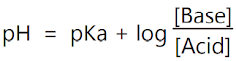equation (10)
• By measuring the pH of the solutions, it is possible to determine the pKa of various drugs.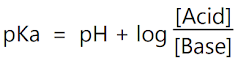equation (11)
• Calculate the A-/HA ratio to provide a buffer with definite pH.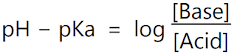equation (12)
• Calculation of the HA/A-ratio is required to produce a buffer of a specified pH.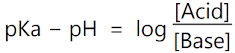equation (13)
• To calculate the pH changes caused by a change in acidity or base in a buffer solution.
• The number of ions in a solution or the number of unions therein.

## Buffer capacity in general

Buffer capacity refers to the ability of a solution to resist rapid changes in pH by absorbing or removing ions such as H+ and OH-. We can change the pH of a buffer system if we add acid or base. There can be both large and/or small changes. The pH of the original solution and the buffer's ability to resist the pH change will have a significant impact on the outcome.

When an acid or base is added to a buffer, the pH is unlikely to change, which causes the buffer to be consumed. Up to the point where the buffer is completely absorbed, the pH does not drastically change. With the depletion of the buffer, the pH will be more prone to change.

Alternatively, buffer capacity can also be simply described as the resistance of the buffer solution to pH changes when H+ or OH- ions are added. As well as buffer capacity, acid neutralizing capacity, or alkalinity capacity are also terms used for this concept. The buffer capacity (β) of a solution can be defined mathematically by dividing the pH difference caused by adding an acid or a base by the moles or grams of an acid or base needed. It can be expressed as follows:

β=ΔB/ΔpH

It is usually the concentration of the species in a buffered solution that determines buffer capacity. A highly concentrated buffer solution will have a larger capacity to buffer. Titration usually determines how much buffer is available.
Get subject wise printable pdf documents

Ankur Choudhary is India's first professional pharmaceutical blogger, author and founder of pharmaguideline.com, a widely-read pharmaceutical blog since 2008. Sign-up for the free email updates for your daily dose of pharmaceutical tips.

## Popular Categories

QA SOPs QC SOPs Micro SOPs HVAC Production SOPs Stores SOPs Checklists Maintenance SOPs HPLC Sterile GLP Validation Protocols Water System GDP Regulatory Maintenance Calibration Warning Letters Education B.Pharmacy
Video Tutorials

Submit Guest Posts

## DOCUMENTS

PHARMACEUTICAL DOCUMENTS

Editable Pharmaceutical Documents in MS-Word Format. Ready to use SOPs, Protocols, Master Plans, Manuals and more...

View